# 真实感海洋的绘制（三）：水面的渲染

Published:

## 基本原理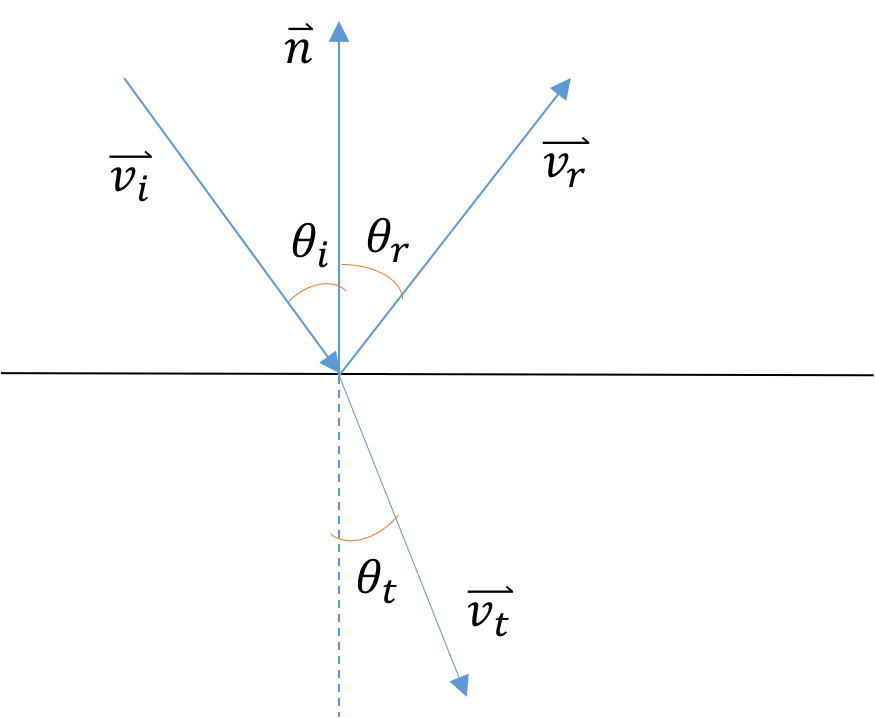$n_i\sin\theta_i=n_t\sin\theta_t$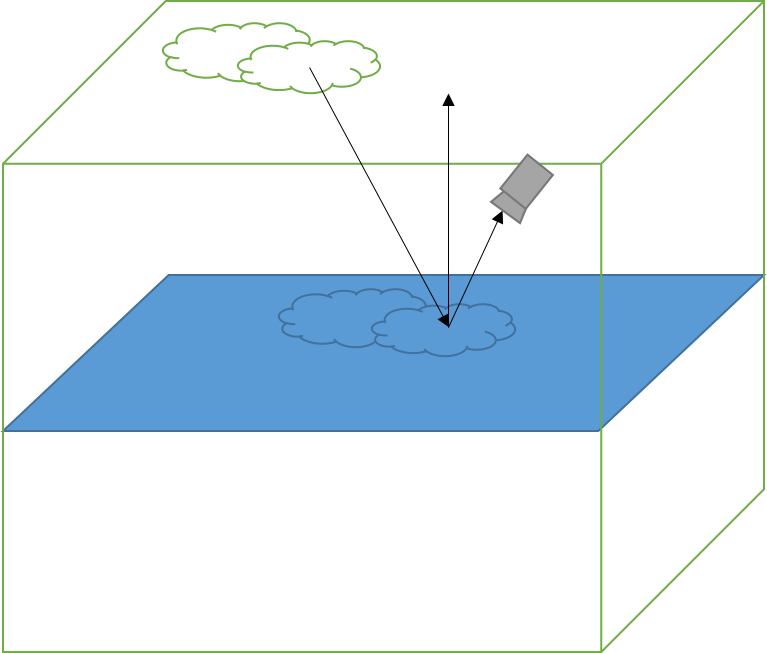$R=\frac{1}{2}\{\frac{\sin^2(\theta_t-\theta_i)}{\sin^2(\theta_t+\theta_i)}+\frac{\tan^2(\theta_t-\theta_i)}{\tan^2(\theta_t+\theta_i)}\}$

$Color = R * Color_{incident} + (1 - R) * Color_{deepWater}$

## 一些美化

$Color = (1 - F(d)) * Color_{object} + F(d) * Color_{fog}$

$F(d)=1 - e^{-cd}$

## 实现结果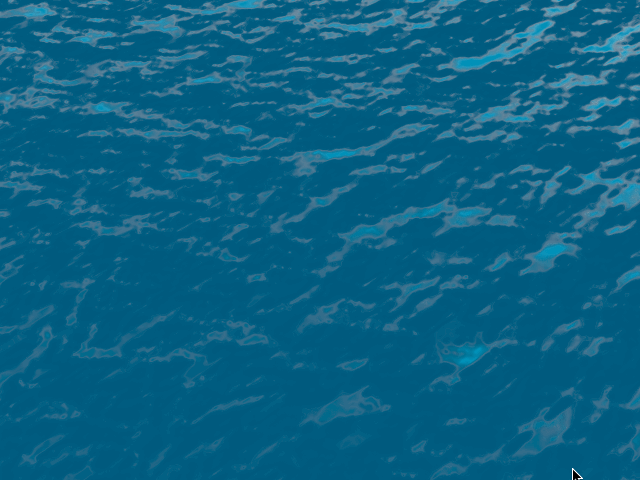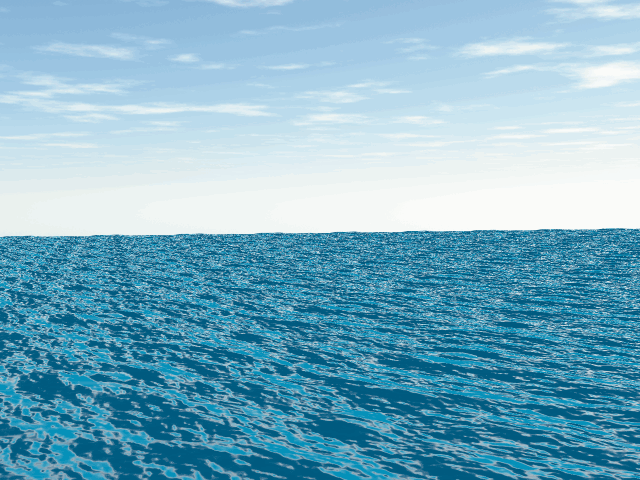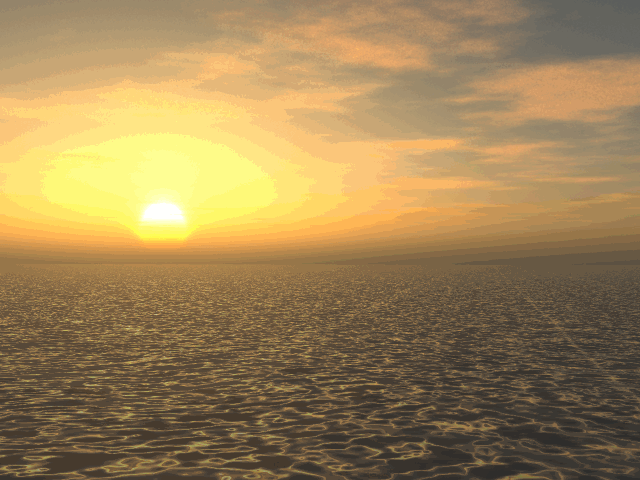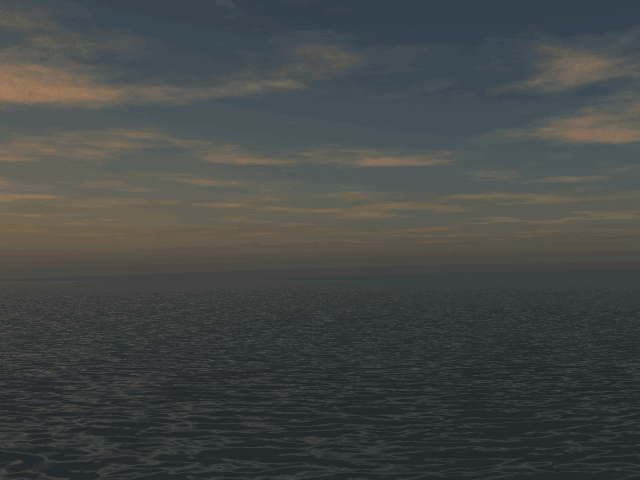## 参考文献

1. Tessendorf, Jerry. Simulating Ocean Water. In SIGGRAPH 2002 Course Notes #9 (Simulating Nature: Realistic and Interactive Techniques), ACM Press.
2. Johanson, Clase. Real-time water rendering - Introducing the projected grid concept. Master of Science Thesis. Lund University
3. Toman, Wojciech. Rendering Water as a Post-process Effect. https://www.gamedev.net/articles/programming/graphics/rendering-water-as-a-post-process-effect-r2642/

Tags: